Courses

# Test: Electrical And Electronic Measurements- 3

## 10 Questions MCQ Test Mock Test Series for Electrical Engineering (EE) GATE 2020 | Test: Electrical And Electronic Measurements- 3

Description
This mock test of Test: Electrical And Electronic Measurements- 3 for Electrical Engineering (EE) helps you for every Electrical Engineering (EE) entrance exam. This contains 10 Multiple Choice Questions for Electrical Engineering (EE) Test: Electrical And Electronic Measurements- 3 (mcq) to study with solutions a complete question bank. The solved questions answers in this Test: Electrical And Electronic Measurements- 3 quiz give you a good mix of easy questions and tough questions. Electrical Engineering (EE) students definitely take this Test: Electrical And Electronic Measurements- 3 exercise for a better result in the exam. You can find other Test: Electrical And Electronic Measurements- 3 extra questions, long questions & short questions for Electrical Engineering (EE) on EduRev as well by searching above.
QUESTION: 1

### A 3 phase 500 V motor load has a power factor of 0.5. Two wattmeter’s connected to measure the input. They show the input to be 36 kW. The readings of both the instruments are.

Solution: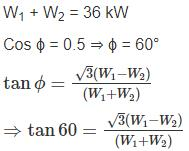⇒ W1 – W2 = 36
⇒ W1 = 36 kW, W2 = 0 kW

*Answer can only contain numeric values
QUESTION: 2

### Two wattmeter’s are connected to measure the power consumed by a 3-phase balanced load one of the wattmeter’s reads 1400 W and the other is 800 W. The power factor of the load is when both the readings are positive.

Solution:

P1 = 1400 W, P2 = 800 W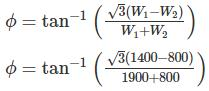= 25.28°
Power factor, cos ϕ = cos 25.28° = 0.90

QUESTION: 3

### In a particular test, the readings of two watt meters are 5 kW, 2 kW. Then the total power and power factor are, if second wattmeter connections are reversed

Solution:

W1 = 5kW,W2 = 2kW
Total power, P = W1 − W2 = 3kW
Power factor is,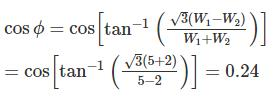*Answer can only contain numeric values
QUESTION: 4

Calculate the magnitude of line current in the circuit shown in figure.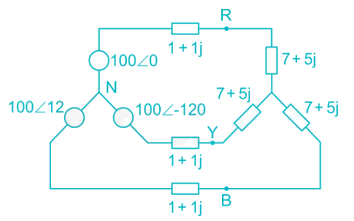Solution:

Impedance/ph = 1 + 1j + 7 + 5j = (8 + 6j)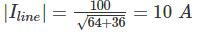QUESTION: 5

In the balanced three phase circuit shown in figure, the current coil of the wattmeter is connected in line aA, and the potential coil of the wattmeter us connected across lines b and c. The phase sequence is positive and the load power factor is 0.8 lag. The wattmeter reading will be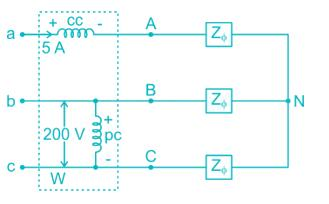Solution:

Current through current coil = Ia
Voltage across the pressure coil = Vbc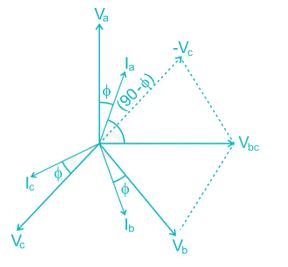Wattmeter reading = Vbc Ia cos(90 - ϕ)
= Vbc Ia sin ϕ
= (200) (5) (0.6) = 600 W

QUESTION: 6

A 5A, 110V electrodynamic type wattmeter has a scale having 110 divisions. Its pressure coil is fed by a voltage of  [110√2cos(314t) + √2sin(942t)]  V and is current coil carries a current of [5√2cos(314t + 60) + 2√2sin(628t + 90) + √2cos(642t + 90)] A. The needle of the wattmeter will move to

Solution:

The average power read by the meter is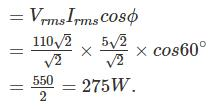Full scale reading of wattmeter = 110 × 5 = 550 W
Scale having 110 divisions for 550 W reading.
Hence number of divisions required for 275 W
= 110/2 = 55

*Answer can only contain numeric values
QUESTION: 7

The constant of the shunt to extend the range 0-10 A moving iron ammeter to 0-100 A, the instrument constant are R = 0.18 Ω and L = 180 μH. If the shunt is made non-inductive and the combination is correct on dc. Find the full-scale error (in percentage) at 50 Hz.

Solution:

Multiplying power of shut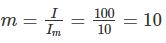∴ Resistance of shunt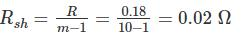Time constant of shunt and meter should be equal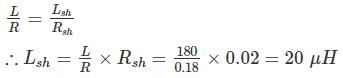The current through the meter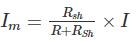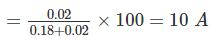With 50 Hz the current through the meter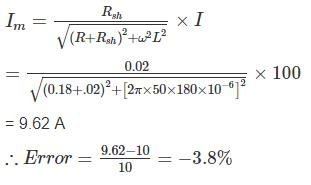*Answer can only contain numeric values
QUESTION: 8

The reading of a dynamometer – type wattmeter with pressure coil phase angle of 2° is 700 watts, when it is used to measure power of a single phase inductive load supplied by 240 V single phase ac. source. when the wattmeter is replaced by a second wattmeter with a phase angle of 1° for the pressure coil circuit, a reading of 620 watts is obtained. Assuming all errors of the wattmeter, except those due to pressure coil inductance are neglected. The actual power is ____________(in W)

Solution: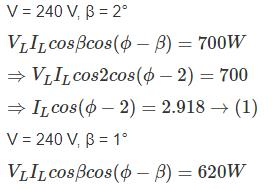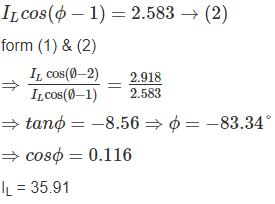True power = VLILcosϕ = 240 × 35.91 × 0.116
= 999.9 W

QUESTION: 9

A wattmeter reads 5.54 kW when its current coil is connected in the red phase and its voltage coil is connected between the neutral and red phase of a symmetrical three phase system supplying a balanced load of 30 A at 400 V. What will be the reading of the instrument if the current coil remains unchanged and voltage coil be connected between blue and yellow phases. The phase sequence is RYB.

Solution:

Let V and I be the phase voltage and phase current respectively.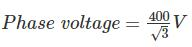Phase current = 30 A
In the first case, the wattmeter measures the power in one phase.
VI cos ϕ = 5.54 × 103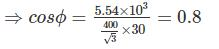When the current coil is connected in the red phase and the pressure coil circuit is connected across the yellow and blue phase, the reading of wattmeter is,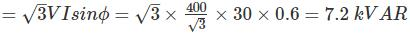*Answer can only contain numeric values
QUESTION: 10

The balanced three phase load shown in figure is fed form a balanced, positive sequence, there phase Y-connected source. The impedance of the line connecting the source to the load is negligible the line to neutral voltage of the source is 7200 V. Find the reading of the wattmeter in kW.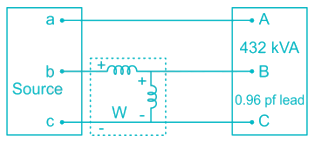Solution: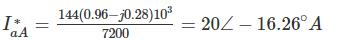VBN = 7200 ∠-120°V
VCN = 7200 ∠120°V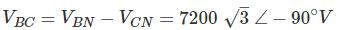IbB = 20 ∠-103.74°A
W = VIcosϕ = (7200)(√3)(20)cos(−90+103.74)
= 242.28 kW